Function Repository Resource:

Compute the hypergraph with a specified adjacency tensor

Contributed by: Jonathan Gorard

## Details and Options

An adjacency tensor is a generalization of the concept of an adjacency matrix from graphs to hypergraphs, in which hyperedges may be of arbitrary arity.
The arity of the hyperedges in the hypergraph is equal to the rank of the adjacency tensor.
The number of vertices will be equal to n, where the dimensions of the adjacency tensor are n×n××n.
ResourceFunction["AdjacencyHypergraph"] accepts adjacency tensors specified both as SparseArray objects, and as ordinary nested lists.
The number of hyperedges of the form {vi,vj,,vk} is specified by the entry aij…k of the adjacency tensor.
The number of self-loops for the vertex vi is given by the diagonal entry aii…i.
 "OrderedHyperedges" Automatic whether to treat hyperedges as being ordered (directed)
The following settings for "OrderedHyperedges" can be used in ResourceFunction["AdjacencyHypergraph"]:
 Automatic construct an orderless hypergraph if and only if the adjacency tensor is symmetric across all indices True construct an ordered hypergraph False construct an orderless hypergraph
When the adjacency tensor is of rank 2, the output of ResourceFunction["AdjacencyHypergraph"] is identical to that of AdjacencyGraph.

## Examples

### Basic Examples (3)

Construct an ordered hypergraph automatically from an asymmetric adjacency tensor:

 In:=Out=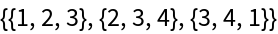Construct an orderless hypergraph automatically from a symmetric adjacency tensor:

 In:=Out=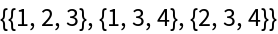Treat the hypergraph as being ordered instead:

 In:=Out=Construct an ordered hypergraph of arity 5 automatically from an asymmetric adjacency tensor specified as a SparseArray:

 In:=Out=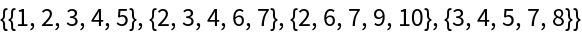### Scope (7)

 In:=Out=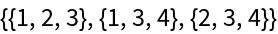In:=Out=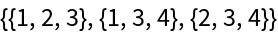In:=Out=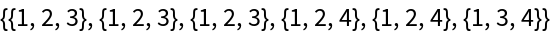When the rank of the adjacency tensor is equal to 2, the output of AdjacencyHypergraph is identical to the output of AdjacencyGraph:

 In:=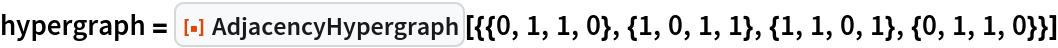Out=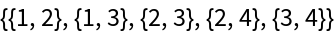In:=Out=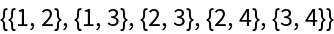In:=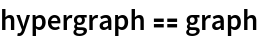Out=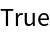When the adjacency tensor is symmetric across all indices, the hypergraph is automatically orderless:

 In:=Out=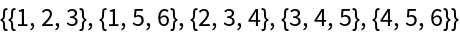In:=Out=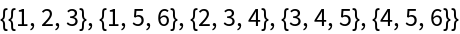In:=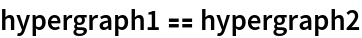Out=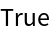When the adjacency tensor is asymmetric across any pair of indices, the hypergraph is automatically ordered:

 In:=Out=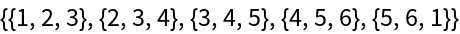In:=Out=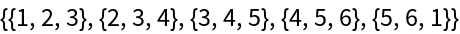In:=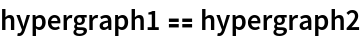Out=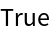Diagonal entries of the adjacency tensor specify self-loops in the hypergraph:

 In:=Out=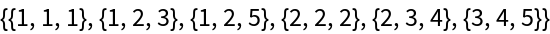Adjacency tensors can be of arbitrary rank:

 In:=Out=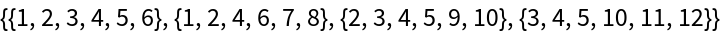### Options (3)

By default ("OrderedHyperedges"Automatic), all hyperedges are treated as orderless (i.e. undirected) if the adjacency tensor is symmetric across all indices:

 In:=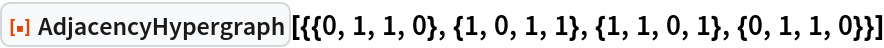Out=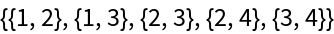Use "OrderedHyperedges"True to treat hyperedges as ordered (i.e. directed):

 In:=Out=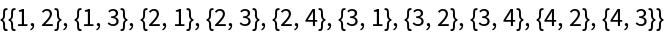Conversely, all hyperedges are treated as ordered (i.e. directed) if the adjacency tensor is asymmetric across any pair of indices:

 In:=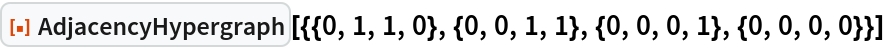Out=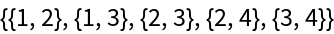Jonathan Gorard

## Version History

• 1.0.0 – 17 March 2021